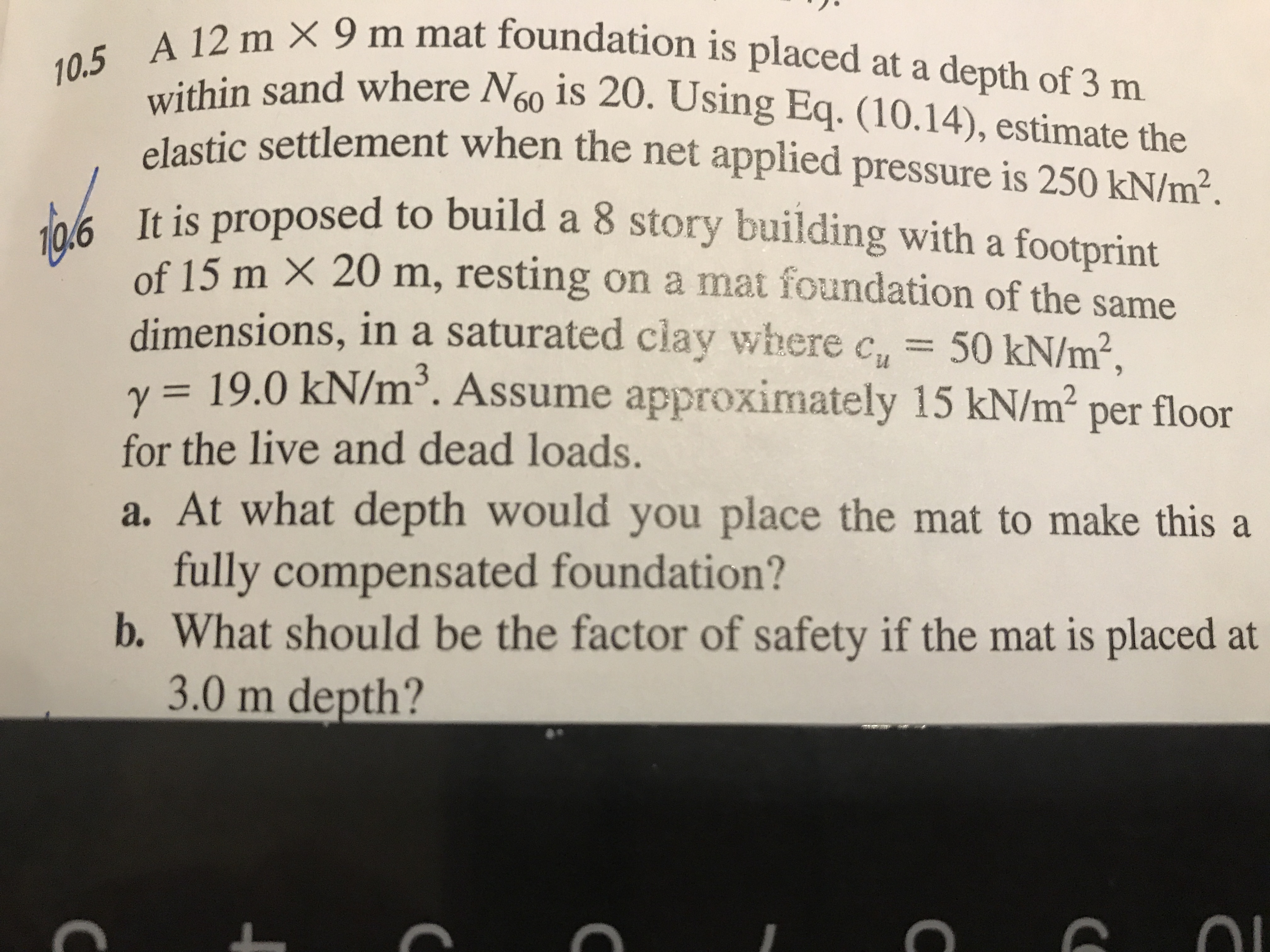# 10.5 A 12 m × 9 m mat foundation is placed at a depth of 3 mwithin sand where N6o is 20. Using Eq. (10.14), estimate theelastic settlement when the net applied pressure is 250 kN/m².It is proposed to build a 8 story building with a footprintof 15 m X 20 m, resting on a mat foundation of the samedimensions, in a saturated clay where c,,v = 19.0 kN/m³. Assume approximately 15 kN/m2 per floor50KN/m²,for the live and dead loads.a. At what depth would you place the mat to make this afully compensated foundation?b. What should be the factor of safety if the mat is placed at3.0 m depth?

Question
17 views

10.6help_outlineImage Transcriptionclose10.5 A 12 m × 9 m mat foundation is placed at a depth of 3 m within sand where N6o is 20. Using Eq. (10.14), estimate the elastic settlement when the net applied pressure is 250 kN/m². It is proposed to build a 8 story building with a footprint of 15 m X 20 m, resting on a mat foundation of the same dimensions, in a saturated clay where c,, v = 19.0 kN/m³. Assume approximately 15 kN/m2 per floor 50KN/m², for the live and dead loads. a. At what depth would you place the mat to make this a fully compensated foundation? b. What should be the factor of safety if the mat is placed at 3.0 m depth? fullscreen
check_circle

Step 1

Given data:

The length of the foundation is 20 m.

The breadth of the foundation is 15 m.

The cohesion of soil is cu =50 kN/m^3.

The unit weight of soil is 50 kN/m^3.

Uniformly distributed load is w =15 kN/m^2.

The number of story’s in building n=8.

a.

The area of foundation is given by,

Step 2

Plugging values,

Step 3

### Want to see the full answer?

See Solution

#### Want to see this answer and more?

Solutions are written by subject experts who are available 24/7. Questions are typically answered within 1 hour.*

See Solution
*Response times may vary by subject and question.
Tagged in

### Geotechnical Engineering